# QtiPlot - Data Analysis and Scientific Visualisation

## Analytical FunctionsA 2D user defined function created in QtiPlot using the analytical expression:

Y = X2 + X + 1

The function is drawn using a scatter plot style.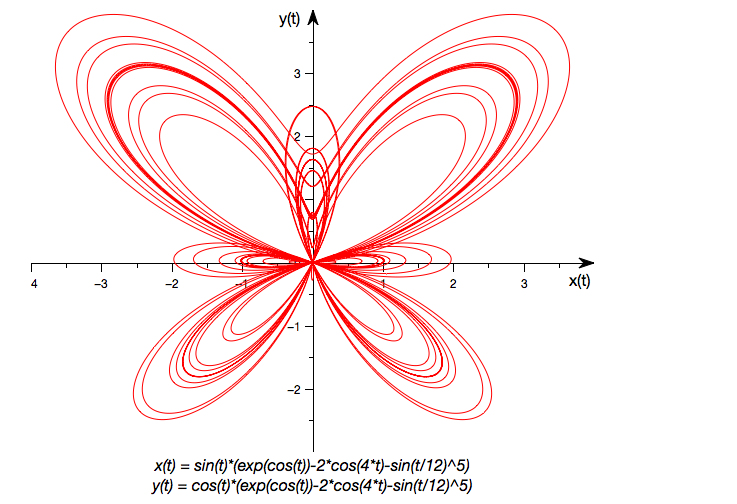A 2D parametric function plot representing a butterfly. It can be easily created in QtiPlot using the parametric equations bellow:

X(t)=sin(t)[ecos(t)-2cos(4t)-sin5(t/12)]

Y(t)=cos(t)[ecos(t)-2cos(4t)-sin5(t/12)]

## Multi-axis GraphsQtiPlot can create double Y axis graphs using a single plot layer. Left and right Y axes may display different scale ranges.

The double Y axis graph requires a selection of at least two Y columns (or a range from at least two columns). The last Y column in the selection range is attached to the right axis of the plot layer.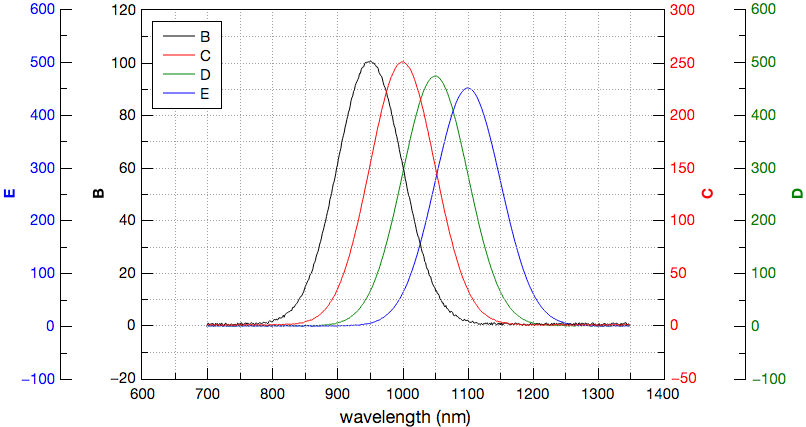By default, QtiPlot can create graphs with up to four Y axes, using two plot layers with overlapping drawing canvases.

The left and right Y axes of the second plot layer are displayed using a horizontal offset with respect to the drawing canvas.

## Line Plots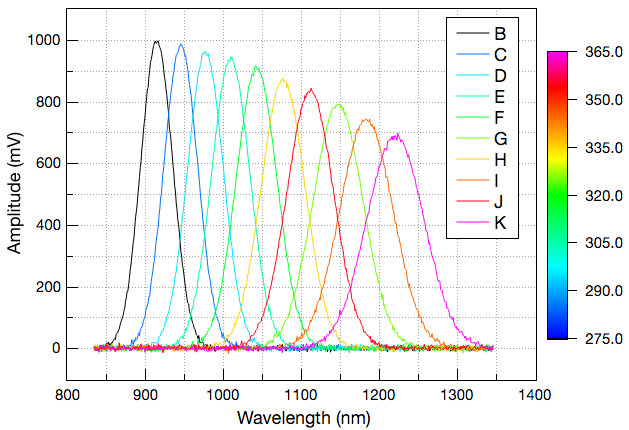The colormapped lines plot displays each data set as a line curve. Existing column comments are treated as Z values if they are valid floating point values. If there are no valid column comments, the column indices define the Z values. The colors of the curves are mapped to these Z values and a color scale legend is added at the right side of the plot layer.The area plot is a line graph having the area below it filled with a coloured brush.

By default, the baseline for the area is the Y = 0 axis. It is possible to define a different baseline value via the plot details dialog.

## Stacked Curves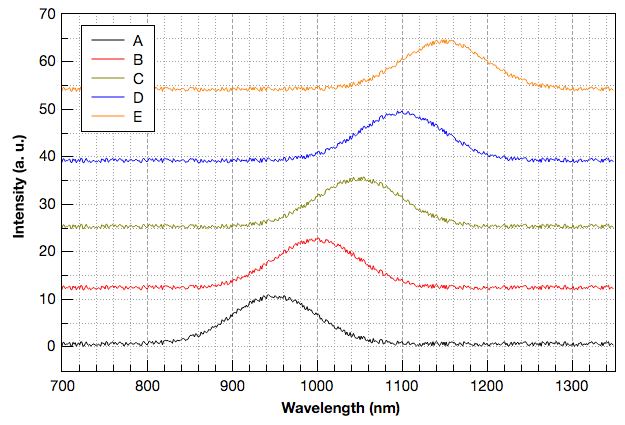The stacked lines plot type draws each data column as a separate curve which has a vertical offset from the previous one. This offset setting that can be modified in the Stack tab of the Plot Details dialog.

The distances between each curve can be adjusted using this offset feature, thus eliminating the need to change the original data. Using this feature prevents the curves from overlapping and ensures that each curve can be viewed clearly.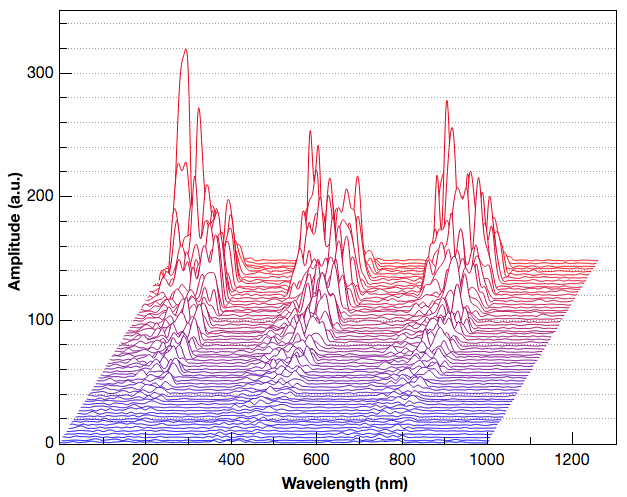For this kind of graph each data column is drawn as a separate line curve which is offset from the previous one in both X and Y directions, creating the so-called waterfall plot effect.

## Bubble Charts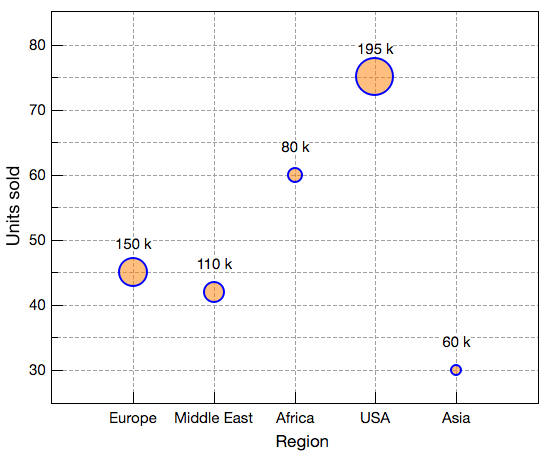A bubble chart draws the selected data columns using indexed sizes for the symbols. You need to select at least two Y columns for this type of plot. The first Y column in the selection is used for the positions of the plot symbols and the next one determines their sizes.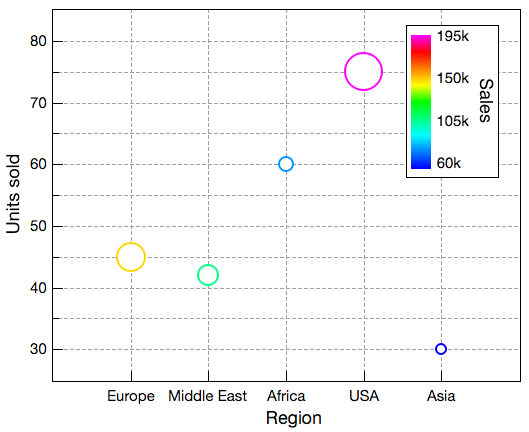The color mapped bubble chart uses a Y data column to control the colors of the symbols. QtiPlot creates evenly sized ranges of values between the minimum and maximum values in this Y column and then associates a color with each range of values.

Optionally, a color scale may be added to this type of graph, like in this example, in order to display the color mapping information.

## Column & Bar Graphs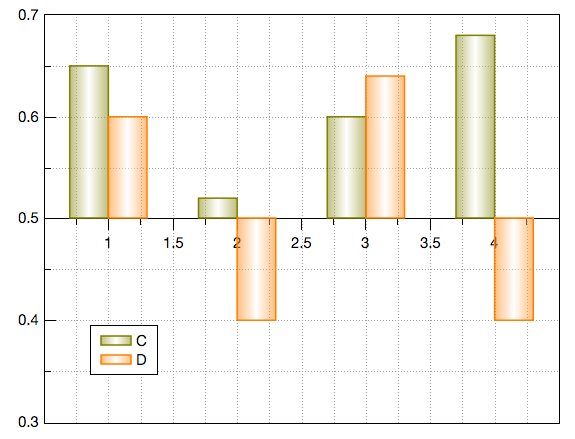A bar chart is a type of graph that presents categorical data with rectangular bars with heights or lengths proportional to the values that they represent. The bars can be plotted vertically or horizontally. A vertical bar chart is sometimes called a column chart.

By default, the baseline for the columns is the Y = 0 axis. It is possible to define a different baseline value via the plot details dialog.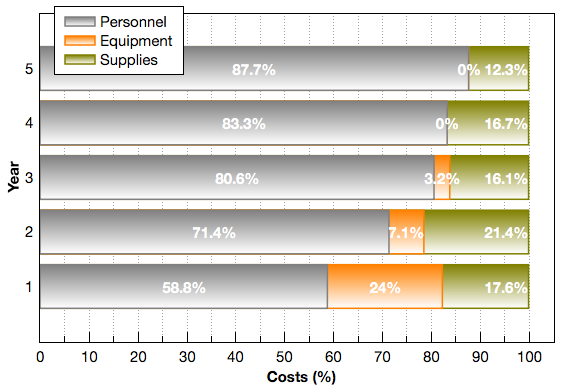The 100% Stack Bar plot compares the percentage that each value contributes to a total across categories.

You can use a 100% stacked bar plot when you have several data sets and you want to emphasize the contributions to the whole, especially if the total is the same for each category.

## Pie Charts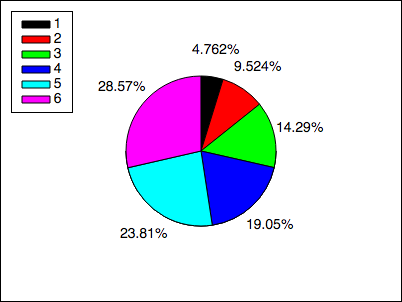This type of graph can be created using the 2D BW Pie Chart command from the plot menu.

By default QtiPlot draws a 2D black and white pie chart using the selected column in the active table window (only one column allowed).

The aspect of the pie chart (colors, fill pattern, line width and pen style) can be easily customized via a double-click on the drawing area which opens the plot details dialog.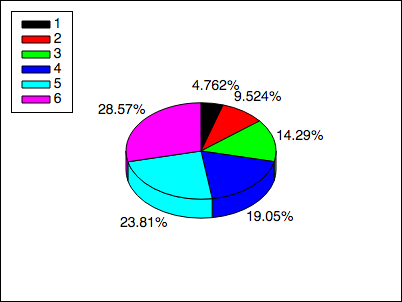This type of graph can be created using the 3D Color Pie Chart command from the plot menu.

QtiPlot draws a pseudo 3D colored pie chart using the selected column in the active table window (only one column allowed).

The 3D geometry settings of the pie chart can be easily customized via a double-click on the drawing area which opens the plot details dialog.

## Contour & HeatmapA contour plot is a graphical technique for representing three-dimensional surfaces in the XY plane using contour lines. A contour line for a function of two variables (X and Y) is a curve connecting points where the function has the same particular Z value.

The aspect of the contour lines can be fully customized in terms of width, color and pen style.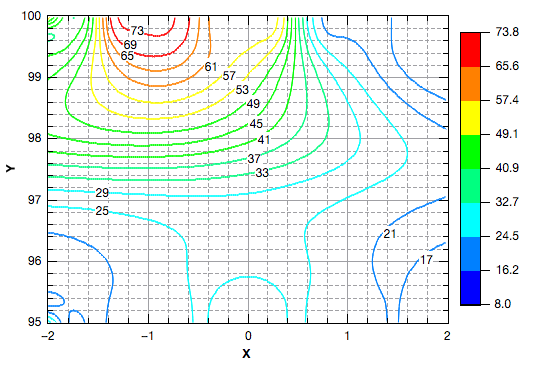This type of graph is a contour plot for which the colors of the contour lines are mapped to the Z scale using a rainbow palette.

Contour labels are displayed for all levels by default. The aspect of these labels can be fully customized in terms of font, color and position.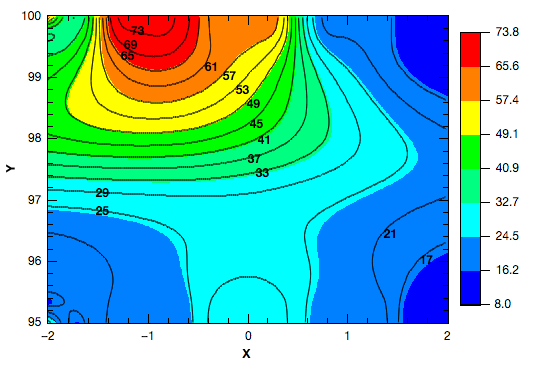Color filled contour plots are drawn using a default rainbow palette. The settings of the color map may be fully customized by clicking on the plotting area, which activates the plot details dialog.A heatmap is a type of graph very similar to the color filled contour plot with the following differences: the contour lines are not drawn, there are no minor ticks and a three color based map (blue, white, red) is used instead of the default rainbow palette.

The color map settings can be changed by double-clicking on the plot area: this opens the plot details dialog.

## Vector Plots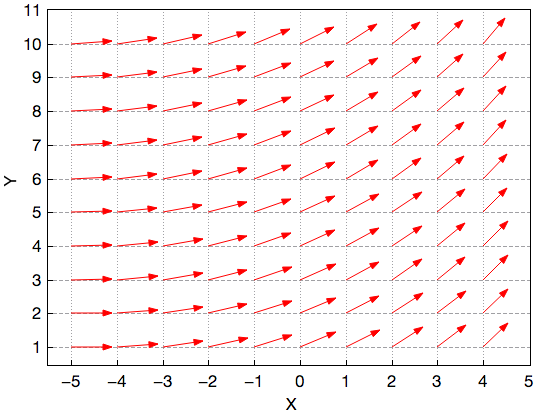The XYXY vectors plot requires four input data columns. The first two columns give the coordinates of the starting points of the vectors, the last two columns give the coordinates of the end points.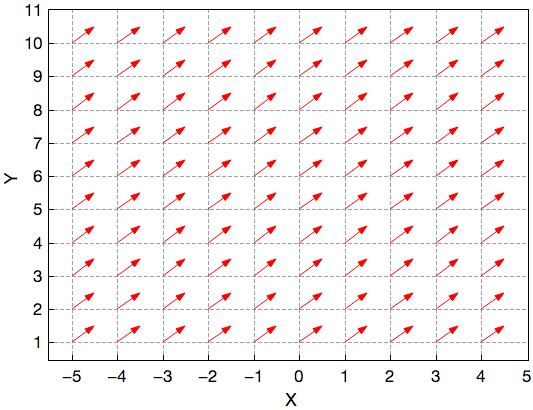The XYAM vectors plot also needs four input data columns: the first two columns define the origin of the vectors, while the last two columns give the angles (in radians) and the magnitudes of the vectors.

It is also possible to define constant angle and magnitude values like in this graph which draws an uniform vector field with a 45 degrees orientation.

## Statistical Graphs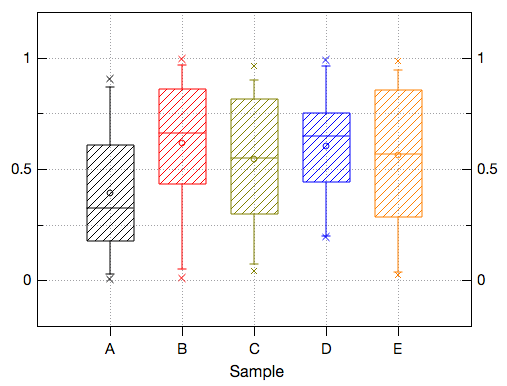The box plots are used to give a graphical representation of some of the classical parameters of a frequency distribution, such as the mean, the minimum and maximum values, the position of the 95 and 5 percentiles, etc.

The choice of the statistical parameters and the graphical parameters can be modified with the plot details dialog.An interval plot is used to compare groups similar to a box plot. It displays the confidence interval for the mean of the data. Typically, a 95% confidence interval is used but any other confidence level can be specified as well.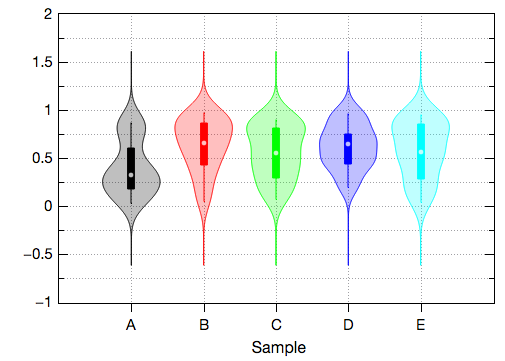A violin plot is similar to a box plot, with the addition of a rotated kernel density plot on each side.

A violin plot is more informative than a plain box plot. While a box plot only shows summary statistics such as mean/median and interquartile ranges, the violin plot shows the full distribution of the data. The difference is particularly useful when the data distribution is multimodal (more than one peak). In this case a violin plot shows the presence of different peaks, their position and relative amplitude.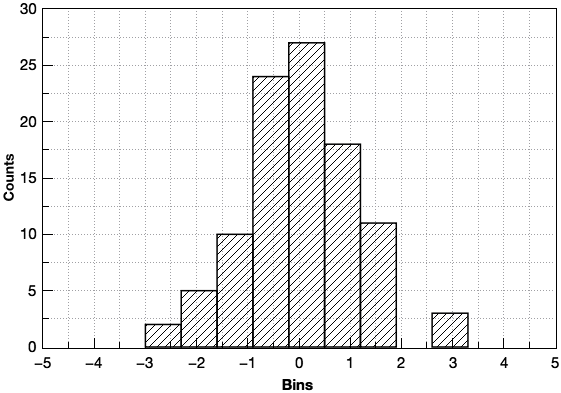The histogram chart draws a frequency distribution that is computed from the data. The default binning uses 10 steps between the maximum and the minimum values. Binning behavior can be customized via the Histogram Data dialog.

It is possible to overlay one of the following pseudo distribution curves on the binned data: Normal, Lognormal, Poisson, Exponential, Laplace, Lorentz, Weibull, Kernel Smooth, Gamma or Binomial. These distribution curves are not the result of a fit operation performed on the binned data. QtiPlot simply determines the data mean, then overlays the curve so that the means coincide. In the case of a two parameter distribution, the second parameter taken into account is the standard deviation of the binned data.The Histogram + Probabilities plot creates a frequency histogram of the selected data column in the active table window. Additionally, it plots a cumulative sum of observations using a vertical steps style.

The cumulative percent curve is linked to the right Y axis of the graph layer, while the histogram is linked to the left Y axis.The stacked histograms graph creates vertically stacked plot layers displaying the histograms of the selected data columns (one histogram per layer).The purpose of a Pareto chart is to highlight the most important among a set of factors.

In quality control it is often used to display the most common sources of defects, the highest occurring type of defect or the most frequent reasons for customer complaints, like in this example.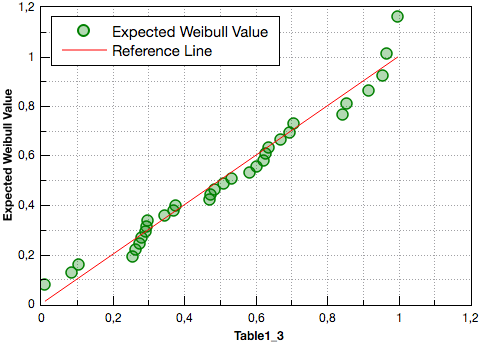The Q-Q (quantile-quantile) plot is a graphical method for comparing two probability distributions by plotting their quantiles against each other. If the two distributions being compared are similar, the points in the Q-Q plot will approximately lie on the line y = x. If the distributions are linearly related, the points in the Q-Q plot will approximately lie on a line, but not necessarily on the line y = x.

There are five distributions available in QtiPlot for Q-Q plots: Normal, Lognormal, Exponential, Weibull and Gamma.